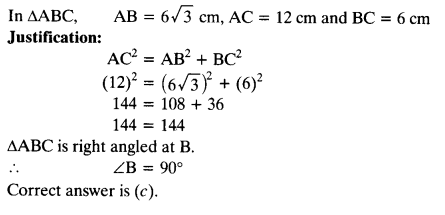Question 1.
Sides of triangles are given below. Determine which of them are right triangles. In case of a right triangle, write the length of its hypotenuse.
(i) 7 cm, 24 cm, 25 cm (ii) 3 cm, 8 cm, 6 cm
(iii) 50 cm, 80 cm, 100 cm (iv) 13 cm, 12 cm, 5 cm
Solution:
(i) 7 cm, 24 cm,-25 cm
(7)2 + (24)2 = 49 + 576 = 625 = (25)2 = 25
∴ The given sides make a right angled triangle with hypotenuse 25 cm

(ii) 3 cm, 8 cm, 6 cm

(8)2 = 64
(3)2 + (6)2 = 9 + 36 = 45
64 ≠ 45
The square of larger side is not equal to the sum of squares of other two sides.
∴ The given triangle is not a right angled.

(iii)
50 cm, 80 cm, 100 cm
(100)2= 10000
(80)2 + (50)2 = 6400 + 2500
= 8900
The square of larger side is not equal to the sum of squares of other two sides.
∴The given triangle is not a right angled.

(iv)
13 cm, 12 cm, 5 cm
(13)2 = 169
(12)2 + (5)2= 144 + 25 = 169
= (13)2 = 13
Sides make a right angled triangle with hypotenuse 13 cm.

Question 2.
PQR is a triangle right angled at P and M is a point on QR such that PM ⊥ QR. Show that PM2 = QM • MR.Solution:
In right angled ∆QPR,
∠P = 90°, PM ⊥ QR
∴ ∆PMQ ~ ∆RMP
[If ⊥ is drawn from the vertex of right angle to the hypotenuse then triangles on both sides of perpendicular are similar to each other, and to whole triangle]

⇒ [Corresponding sides of similar
⇒ PM x MP = RM x MQ ⇒ PM2 = QM.MR

Question 3.
In the given figure, ABD is a triangle right angled at A and AC i. BD. Show that
(i) AB2 = BC.BD
(ii) AC2 = BC.DC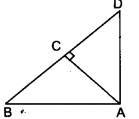Solution: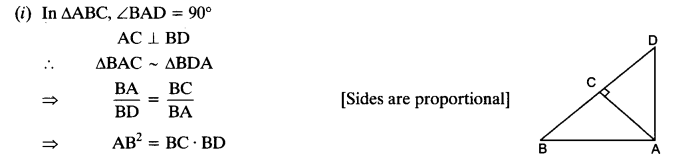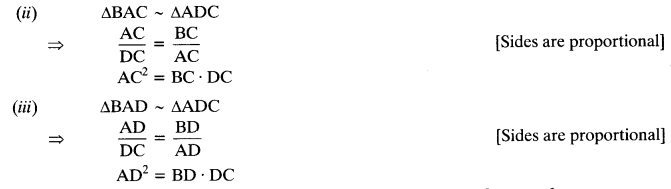Question 4.
ABC is an isosceles triangle right angled at C. Prove that AB2 = 2AC2.
Solution:
Given: In ∆ABC, ∠C = 90° and AC = BC
To Prove: AB2 = 2AC2
Proof: In ∆ABC,
AB2= BC2 + AC2
AB2 = AC2 + AC2 [Pythagoras theorem]
= 2AC2

Question 5.
ABC is an isosceles triangle with AC = BC. If AB2 = 2AC2 , Prove that ABC is a right triangle.
Solution:Question 6.
ABC is an equilateral triangle of side la. Find each of its altitudes.
Solution:Given: In ∆ABC, AB = BC = AC = 2a
We have to find length of AD
In ∆ABC,
AB = BC = AC = 2a
BD = 12 x 2 a = a
⇒ AD2 = AB2 – BD2= (2a)2 – (a)2 = 4a2– a2= 3a2

Question 7.
Prove that the sum of the squares of the sides of a rhombus is equal to the sum of the squares of its diagonals.
Solution:
Given: ABCD is a rhombus. Diagonals AC and BD intersect at O.
To Prove: AB2+ BC2+ CD2+ DA= AC2+ BD2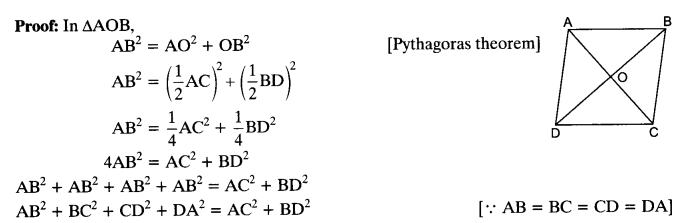Question 8.
In the given figure, O is a point in the interior of a triangle ABC, OD ⊥ BC, OE ⊥ AC and OF ⊥ AB. Show that
(i) OA2 + OB2 + OC2 – OD2 – OE2 – OF2 = AF2 + BD2 + CE2
(ii) AF2 + BD2 + CE2 = AE2 + CD2 + BF2.Solution: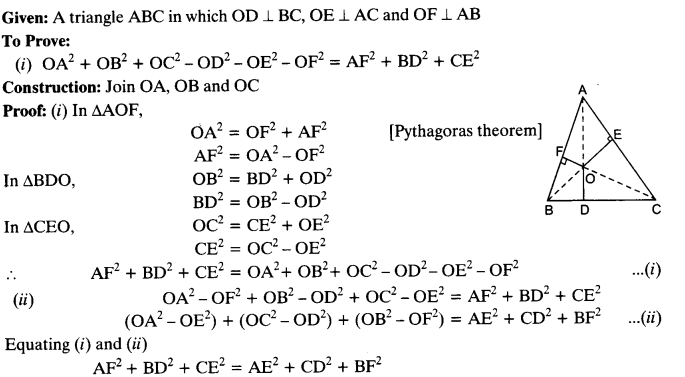Question 9.
A ladder 10 m long reaches a window 8 m above the ground. ind the distance of the foot of the ladder from base of the wall.
Solution: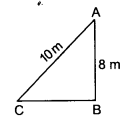Let AC be the ladder of length 10 m and AB = 8 m
In ∆ABC, BC2 + AB2 = AC2
⇒ BC2= AC2 – AB2= (10)2 – (8)2
BC2 = 100-64 – 36 BC = √36 = 6 m
Hence distance of foot of the ladder from base of the wall is 6 m.

Question 10.
A guy wire attached to a vertical pole of height 18 m is 24 m long and has a stake attached to the other end. How far from the base of the pole should the stake be driven so that the wire will be taut?
Solution:Question 11.
An aeroplane leaves an airport and flies due north at a speed of 1000 km per hour. At the same time, another aeroplane leaves the same airport and flies due west at a speed of 1200 km per hour. How far apart will be the two planes after 112 hours?
Solution: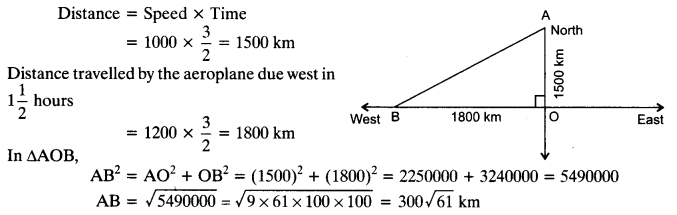Question 12.
Two poles of heights 6 m and 11m stand on a plane ground. If the distance between the feet of the poles is 12 m, find the distance between their tops.
Solution: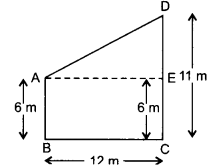Length of poles is 6 m and 11m.
DE = DC – EC = 11m-6m = 5m
In ∆DAE,
AD2 = AE2 + DE2 [ ∵AE = BC]
= (12)2 + (5)2 =144 + 25 = 169

Question 13.
D and E are points on the sides CA and CB respectively of a triangle ABC right angled at C. Prove that AE2 + BD2 = AB2 + DE2.
Solution: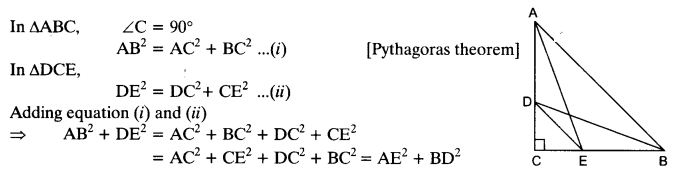Question 14.
The perpendicular from A on side BC of a ∆ABC intersects BC at D such that DB = 3CD (see the figure). Prove that 2AB= 2AC2 + BC2.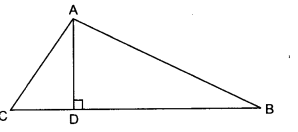Solution: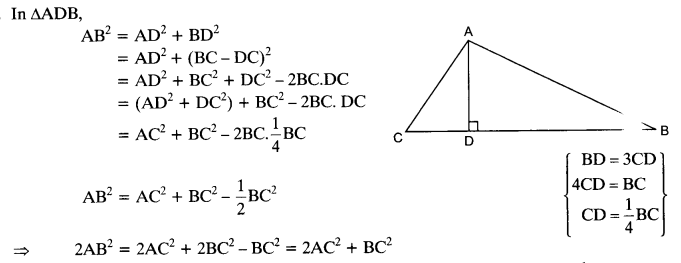Question 15.
In an equilateral triangle ABC, D is a point on side BC, such that BD = 13BC. Prove that 9AD2 = 7AB2.
Solution:Question 16.
In an equilateral triangle, prove that three times the square of one side is equal to four times the square of one of its altitudes.
Solution: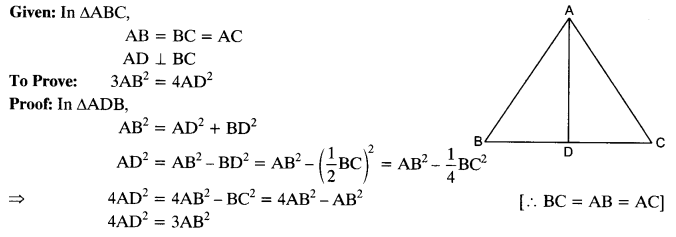Question 17.
Tick the correct answer and justify : In ∆ABC, AB = 6√3 cm, AC = 12 cm and BC = 6 cm. The angle B is:
(a) 120°
(b) 60°
(c) 90°
(d) 45

Solution: